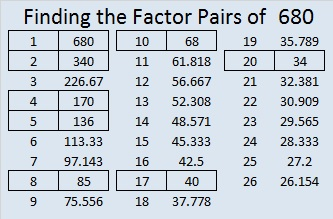# 680 What Would Happen If Ten-Frames Looked Like This?

680 is a number made using only even digits. (There’s much more about 680 at the end of the post.)

Numbers ending in 0, 2, 4, 6, or 8 are even. Numbers ending in 1, 3, 5, 7 or 9 are odd. Those two simple concepts are not always easy for young children to understand.

Sometimes we teach rhymes to children to help them know the difference:

• 0, 2, 4, 6, 8; being EVEN is just great.
• 1, 3, 5, 7, 9; being ODD is just fine.

Still students in early grades struggle with the concepts of odd and even.

Another seemingly simple concept is what pairs of numbers add up to ten. That concept also isn’t as easy for children to understand as adults might think.

Donna Boucher is an elementary school math interventionist with many years experience. Besides many other topics, she is an expert on teaching adding and subtracting to first and second graders. Here are a couple of her tweets with links to her site:

and

Free ten-frame flash cards are available on her site to help students learn addition and subtraction facts. What a powerful way for students to learn! She also has Halloween/Thanksgiving ten-frames for sale at Teachers Pay Teachers.

As I read her post about how to use the ten-frame flash cards I wondered what would happen if we followed her instructions EXACTLY, but the ten-frames looked like this:Children would still learn how to add and subtract, but would they also instinctively learn the difference between odd and even numbers?

Would they figure out for themselves that adding two even numbers or adding two odd numbers ALWAYS makes an even number? Or that adding an odd number and an even number together ALWAYS makes an odd number? Or would changing the ten-frames not make any difference at all? Will the mitten ten-frames only make a difference if the parent/teacher/tutor talks about the odd and even numbers?

I don’t know the answer to those questions, but I think the idea is worth trying. I’ve made Mitten Ten-Frames for all the numbers from 0 to 10. The “empty” frames have outlines of mittens to help children know if a left or a right mitten belongs there. The mitten ten-frames don’t have a second border to guide in cutting them out, so the flashcards might not look as good as Donna Boucher’s, but they should still work as flashcards. Follow Donna Boucher’s instructions exactly. If you use the mitten ten-frames, please add a comment to let me know whether or not they make any difference helping students learn the properties of odd and even numbers.

———————————————————–

Here’s more about the number 680:

1² + 3² + 5² + 7² + 9² + 11² + 13² + 15² = 680.

Because 5, 17, and 85 are some of its factors, 680 is the hypotenuse of four Pythagorean triples. Can you find the greatest common factor of each triple?

• 104-672-680
• 288-616-680
• 320-600-680
• 408-544-680

680 the 15th tetrahedral number. OEIS.org tells us that it is also the smallest tetrahedral number that can be made by adding two other tetrahedral numbers together, specifically the sum of the 10th and the 14th tetrahedral numbers equals this 15th tetrahedral number as shown below:

• (10)(11)(12)/6 = 220
• (14)(15)(16)/6 = 560
• 220 + 560 = 680
• (15)(16)(17)/6 = 680

Finally, here is the factoring information for 680:

• 680 is a composite number.
• Prime factorization: 680 = 2 x 2 x 2 x 5 x 17, which can be written 680 = (2^3) x 5 x 17
• The exponents in the prime factorization are 1, 3, and 1. Adding one to each and multiplying we get (3 + 1)(1 + 1)(1 + 1) = 4 x 2 x 2 = 16. Therefore 680 has exactly 16 factors.
• Factors of 680: 1, 2, 4, 5, 8, 10, 17, 20, 34, 40, 68, 85, 136, 170, 340, 680
• Factor pairs: 680 = 1 x 680, 2 x 340, 4 x 170, 5 x 136, 8 x 85, 10 x 68, 17 x 40, or 20 x 34
• Taking the factor pair with the largest square number factor, we get √680 = (√4)(√170) = 2√170 ≈ 26.0768096.## 2 thoughts on “680 What Would Happen If Ten-Frames Looked Like This?”

1.Paula Beardell Krieg

The ten frame looks like such a good idea. It seems to me that only teachers have the kind of understanding of how children’s minds work to be able to come up with this sort of visual aid.

•ivasallay

So true. A week before I saw those tweets, I helped my granddaughter with some math homework. The 10-frames would have been very helpful to her with that assignment.

This site uses Akismet to reduce spam. Learn how your comment data is processed.